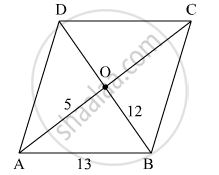Advertisement Remove all ads

# Diagonals of a Parallelogram Intersect Each Other at Point O. If Ao = 5, Bo = 12 and Ab = 13 Then Show that □ Abcd is a Rhombus. - Geometry

Sum

Diagonals of a parallelogram intersect each other at point O. If AO = 5, BO = 12 and AB = 13 then show that
squareABCD is a rhombus.

Advertisement Remove all ads

#### SolutionIn Δ AOB,
AO = 5 cm
OB = 12 cm
AB = 13 cm
5, 12 and 13 form a Pythagorean triplet.
Thus, Δ AOB is a right angle triangle, right angled at O.
ABCD is a parallelogram.
So, diagonals bisect each other.
⇒ AO = OC = 5 cm
Also, OB = OD = 12 cm.
Thus, in parallelogram ABCD, diagonals bisect at right angles.
Hence, ABCD is a rhombus.

Is there an error in this question or solution?
Advertisement Remove all ads

#### APPEARS IN

Balbharati Mathematics 2 Geometry 9th Standard Maharashtra State Board
Chapter 5 Quadrilaterals
Practice Set 5.1 | Q 5 | Page 62
Advertisement Remove all ads
Advertisement Remove all ads
Share
Notifications

View all notifications

Forgot password?